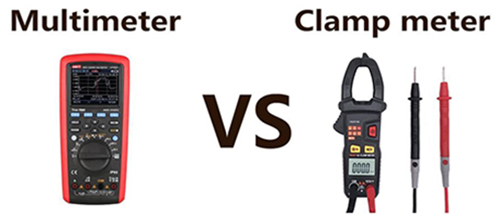Close
(0) Shopping cart
You have no items in your shopping cart.
Shopping Categories
Search

# Clamp Meter VS. Multimeter

### Basic introduction of clamp meter

Clamp meters are divided into mechanical pointer clamp meters and digital clamp meters. Its biggest advantage is that it can measure the current in the line without disconnecting the line to be measured. The current multi-function clamp meter also carries test leads and other voltage resistance gears, and can also be used as a multimeter, and the clamp current meter is also divided into pointer type and digital type, which are roughly the same as the multimeter classification, but the clamp meter head has a transformer, so it is more troublesome to carry.

The most prominent feature of the clamp meter is the caliper that can be opened at the front, which can be easily clipped into the wire to measure the current in the loop, so that the original circuit does not need to be damaged or changed, and a large current can be measured.### Basic introduction of multimeter

A multimeter is a common meter that integrates a voltmeter, an ammeter and an ohmmeter. The multimeter has the advantages of multi-purpose, wide range and convenient use. It is the most commonly used tool in electrical measurement. In electrical maintenance work, it can be used to measure resistance, AC and DC voltage and DC current. Some multimeters can also measure the main parameters of transistors and the capacitance of capacitors. Mastering how to use a multimeter is a basic skill in electrical technology.

Multimeters are divided into pointer and digital automatic multimeters. The pointer type multimeter is a multi-functional measuring instrument with the head as the component, and the measured value is read by the pointer of the head. The measured value of the digital multimeter is displayed directly by the liquid crystal display in the form of numbers, which is easy to read, and some also have a voice prompt function.

When the multimeter measures current, it is necessary to disconnect the circuit under test and connect the multimeter in series to measure the current. The current measurement circuit in the multimeter is measured by converting the current into voltage by means of shunt resistance. The selection of the resistance value of this resistor is also required. If the resistance value is too large, the voltage drop generated when the current passes through the resistance will be large, on the one hand, more voltage will be divided, which will affect the normal operation of the measurement load. On the other hand, the larger the resistor value, the greater the power dissipation generated on it at the same current, which will make the resistor heat up. It can be seen that the multimeter is not allowed to measure large currents for a long time.

Therefore, considering these two issues, the smaller the resistance value, the better. However, the resistance value should not be too small. If the resistance is too small, the voltage drop generated when the current flows will be smaller. In this way, there are certain requirements for the subsequent measurement circuit, because the voltage that is too low needs to be amplified before it can be detected by the circuit. It can be seen that the multimeter is not allowed to measure high current for a long time. For the measurement of high current, the general multimeter is not easy to achieve.

### Differences between clamp meter and multimeter

1. Clamp meter measuring current without a meter pen, as long as the clamp is connected to the working circuit, the reading can be displayed immediately. This is very convenient. So the clamp meter has become the standard equipment for people who often measure current and power.

2. Ordinary multimeters can also measure current, but the meter needs to be connected to the circuit, which belongs to the category of destructive testing. Moreover, the concatenation process is troublesome and time consuming. Therefore, when we measure current, especially large current, we will choose to use a clamp meter for measurement, which is convenient and fast.

3. The clamp meter can continuously detect the current. A multimeter can't do this. If you need to measure AC and DC current frequently, it is recommended to buy a clamp meter. If you do not need to measure the current frequently, buy a multimeter.

4. In terms of price, the current clamp meter adopts the Hall induction principle, which can not only measure AC and DC current, but also is a digital multimeter when the test pen is plugged in, it can also measure parameters such as voltage, resistance, capacitance, frequency ,etc. Its accuracy is no different from that of a digital multimeter, so the clamp meter is relatively more expensive.